# Bolza problem

(diff) ← Older revision | Latest revision (diff) | Newer revision → (diff)

One of the main problems in the classical calculus of variations on the conditions for an extremum, given some constraints on the type of equations; formulated in 1913 by O. Bolza. The problem consists of the minimization of the functional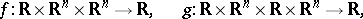in the presence of differential constraints on the type of the equation: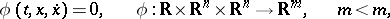and the boundary conditionsIf, the problem is known as the Lagrange problem, if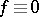and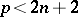it is known as the Mayer problem. A special feature of the Bolza problem is the mixed nature of the functional, which is represented as the sum of an integral functional and a function on the boundary. In principle, the Bolza problem is equivalent to the Lagrange problem, and in fact leads to it if one sets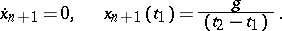It also leads to the Mayer problem if one setswhereThe choice of a given form of the problem, and the choice of the topology within which the problem is then considered, is determined by convenience or by expection in each particular case. In the theory of optimal control the problem is usually considered in the form of Mayer's problem; in the classical calculus of variations it is considered in the form of Lagrange's problem. The most extensively used topology is that of the space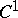of continuously-differentiable functions. To obtain necessary or sufficient conditions for the extremum, smoothness requirements must be imposed on the functionsandinvolved in the definition of the problem and on the mappingsand, as well as requirements concerning the regularity of these mappings, i.e. the matrices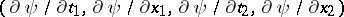and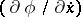must have maximal rankand, respectively. For the vector function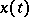to give an extremum in the Bolza problem (and, similarly, in the Lagrange or Mayer problem), it is necessary that is satisfies also the Euler equation and the Weierstrass conditions (for a variational extremum) with respect to the Lagrange function constructed using the data of the problem with Lagrange multipliers, as well as the Jacobi condition and the transversality condition.

The notation employed in the above formulation of the Bolza problem is that used in optimal control theory. In the classical calculus of variations the Bolza problem is formulated using a different notation:How to Cite This Entry:
Bolza problem. Encyclopedia of Mathematics. URL: http://encyclopediaofmath.org/index.php?title=Bolza_problem&oldid=46102
This article was adapted from an original article by I.B. Vapnyarskii (originator), which appeared in Encyclopedia of Mathematics - ISBN 1402006098. See original article